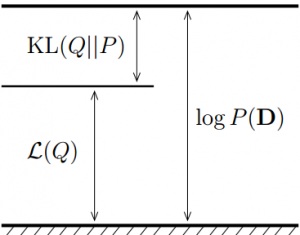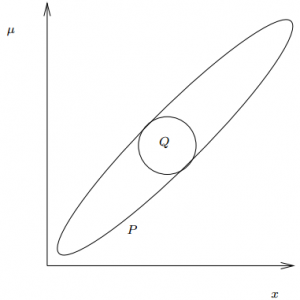# 变分贝叶斯算法理解与推导

## Variational Bayes

Posted by huajh7 on March 6, 2013

## 前言

1. 近似不可观测变量的后验概率，以便通过这些变量作出统计推断。

2. 对一个特定的模型，给出观测变量的边缘似然函数（或称为证据，evidence）的下界。主要用于模型的选择，认为模型的边缘似然值越高，则模型对数据拟合程度越好，该模型产生Data的概率也越高。

## 问题描述

1. 假设存在这样的$Q(Z)$,那么如何度量$Q(Z)$$P(Z \vert D)$之间的差异性 (dissimilarity).

2. 如何得到简单的$Q(Z)$?

## Kullback-Leibler散度

$D_{KL}(p \vert \vert q) = \sum_{x} p(x) \log \frac{p(x)}{q(x)}$.

KL散度有如下性质：

1. ${D_{KL}}(p \vert \vert q) \ne {D_{KL}}(q \vert \vert p)$;
2. ${D_{KL}}(p \vert \vert q) \ge 0$ ，当且仅当$p=q$时为零；
3. 不满足三角不等式。

Q分布与P分布的KL散度为：## 平均场理论（Mean Field Method）

### 平均场方法的合理性

（为保持文章的连贯性，此处先不证明，下文将详细说明）

1. 初始化${Q^{(0)}}(Z_i)$，可随机取；
2. 在第k步，计算${Z_{-i}}$的边缘密度$Q^{[k]}({Z_{-i}} \vert D) \propto \exp \int\limits_{Z_i^*} Q^{[k - 1]}(Z_i \vert D) \log P(Z_i,Z_{-i},D)dZ_i$
3. 计算$Z_i$的边缘密度$Q^{[k]}(Z_i \vert D) \propto \exp \int\limits_{Z_{-i}^*} Q^{[k]}({Z_{-i}} \vert D) \log P(Z_i,{Z_{-i}},D)d{Z_{-i}}$
4. 理论上${Q^{[\infty ]}}(Z_i \vert D)$将会收敛，则反复执行(2), (3)直到$Q(Z_i)$,$Q({Z_{-i}})$稳定，或稳定在某个小范围内。
5. 最后，得 $Q(Z) = Q(Z_i \vert D)Q({Z_{-i}} \vert D)$

### 平均场估计下边缘概率的无意义性 (VB-marginals)### 泛函取极值的必要条件

“当变量函数为$y(x)$时，泛函$J [y]$取极大值”的含义就是：对于极值函数$y(x)$及其“附近”的变量函数$y(x) + \delta y(x)$，恒有$J\left[ {y + \delta y} \right] \le J[y]$;

1. $% $;
2. 有时还要求$% $.

Euler–Lagrange方程

## 问题求解

C为归一化常数$C = \int \exp \left\langle {\ln (Z_i,{Z_{-i}},D)} \right\rangle_{Q_{-i}({Z_{-i}})} d Z_{-i}$ , $Q(Z_i)$为联合概率函数在除$Z_i$本身外的其他划分下的对数期望。又可以写为 $\ln Q_i(Z_i) = {\left\langle {\ln P(Z_i,{Z_{-i}},D)} \right\rangle_{Q_{-i}({Z_{-i}})}} + const$.

## 参考文献

 Smídl, Václav, and Anthony Quinn. The variational Bayes method in signal processing. Springer, 2006.

 Beal, Matthew James. Variational algorithms for approximate Bayesian inference. Diss. University of London, 2003.

 Fox, Charles W., and Stephen J. Roberts. “A tutorial on variational Bayesian inference.” Artificial Intelligence Review 38.2 (2012): 85-95.

 Attias, Hagai. “Inferring parameters and structure of latent variable models by variational Bayes.” Proceedings of the Fifteenth conference on Uncertainty in artificial intelligence. Morgan Kaufmann Publishers Inc., 1999.

 Attias, Hagai. “A variational Bayesian framework for graphical models.”Advances in neural information processing systems 12.1-2 (2000): 209-215.